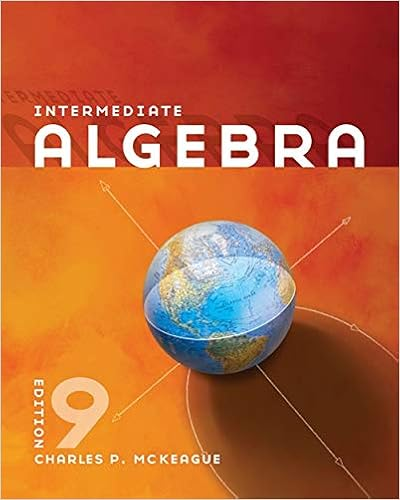# Week 3-Chapter 3 In class Activities A (2).docx - Math 040...

• Homework Help
• 3
• 50% (2) 1 out of 2 people found this document helpful

This preview shows page 1 - 3 out of 3 pages.

##### We have textbook solutions for you!
The document you are viewing contains questions related to this textbook.The document you are viewing contains questions related to this textbook.
Chapter 1 / Exercise 61
Intermediate Algebra
MckeagueExpert Verified
Math 040 : Re-Entry Mathematics Franklin University Week 3 Chapter 3 In class activities Name: Dyl 1. Identify like terms: a. 8b, 17y, -22, 14y, 4xy, 17, -9xy, -9h ( 17y and 14y, 4xy and -9xy, and -22 and 17) 2. Apply the associative property, the commutative property or the distributive property to the given expression and rewrite or simplify the expression. a. a(-4) -4a b. 7 + (5 + p) 12+p c. 3(6x) 18x d. -3(-5q – 2s – 3t) 15q+6s+9t 3.Combine like terms by adding, subtracting or clearing parentheses. a.14a – 5b + 3a – b – 3 17a-4b-3 b.– 3( 2x + 1) – 13 -6x + -16c.– 5 – 11p + 23p – p + 4 1-+11Pd.–( 8w + 5) + 3(w – 15) -5w - 50 4. Identify as an expression or equation. a. 8x – 9 – 7 Expression b. 2( x – 4) – x = 1 Equation c. 24 – 5x + 12 + x Expression 5. Determine whether the given number is a solution to the equation. a. 3y – 2 = 4; 2 Yes b. 39 = – 7p + 4; 5 No c. –z + 8 = 20; 12 No 6. Solve the equations: a. – 4 = y + 18 Y=-22 d. v 10 = – 4 V= 40 b. -8k = 56 1 050315
##### We have textbook solutions for you!
The document you are viewing contains questions related to this textbook.The document you are viewing contains questions related to this textbook.
Chapter 1 / Exercise 61
Intermediate Algebra
MckeagueExpert Verified
Math 040 : Re-Entry Mathematics Franklin University K=-7 e. 3(r – 2) – 2r = – 2 + 6 R=10 c. 11 – 7x + 8x = – 4 – 2 X=-017
•••